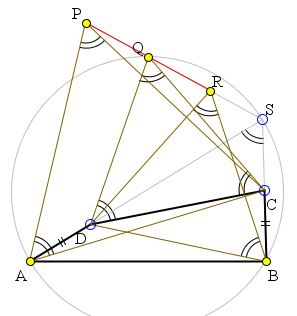## Equilateral Triangles on Segments of Equilic Quadrilateral: What is this about? A Mathematical Droodle

### If you are reading this, your browser is not set to run Java applets. Try IE11 or Safari and declare the site https:///www.cut-the-knot.org as trusted in the Java setup.What if applet does not run?

DiscussionThe applet may suggest the following statement due to Jack Garfunkel [Honsberger]:

If equilateral triangles are drawn on the segments AC, DC, DB (away from AB) of an equilic quadrilateral ABCD, then the three apexes P, Q, R are collinear.

(To remind, a quadrilateral ABCD is called equilic if it has a pair of equal opposite Segments inclined 60° to each other. In the problem, AD = BC.)

### If you are reading this, your browser is not set to run Java applets. Try IE11 or Safari and declare the site https:///www.cut-the-knot.org as trusted in the Java setup.What if applet does not run?

The proof depends on a property of equilic quadrilaterals, viz., ΔAQB is equilateral. Thus a 60° counterclockwise rotation about A carries B to Q. But, because ΔACP is equilateral, this rotation also maps C onto P. It follows that this rotation carries BC onto PQ. In particular, it follows that BC is inclined 60° to PQ and they are equal.

Similarly, a 60° clockwise rotation about B carries AD onto QR so that AD = QR and they are inclined -60° to each other. Thus PQ = QR and they are a continuation of each other. P, Q, R are therefore collinear.

From the diagram it is also obvious that the three points are collinear also with S which is the intersection of AD and BC extended. This property will be proved in a more general setting.

## References

1. R. Honsberger, Mathematical Gems III, MAA, 1985, pp. 32-35